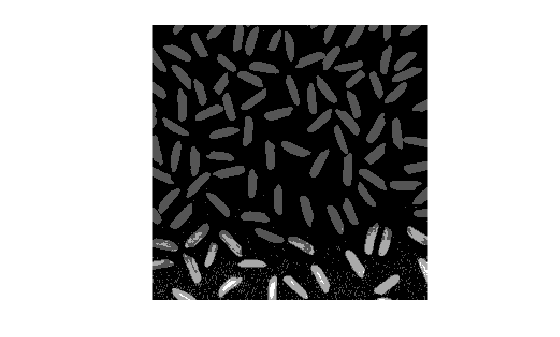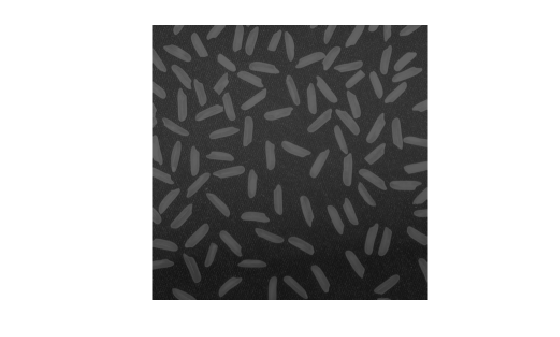imdivide

Divide one image into another or divide image by constant

Description

example

Z = imdivide(X,Y) divides each element in the array X by the corresponding element in array Y and returns the result in the corresponding element of the output array Z.

Examples

collapse all

This example shows how to divide two uint8 arrays.

X = uint8([ 255 0 75; 44 225 100]);
Y = uint8([ 50 50 50; 50 50 50 ]);

Divide each element in X by the corresponding element in Y. Note that fractional values greater than or equal to 0.5 are rounded up to the nearest integer.

Z = imdivide(X,Y)
Z = 2x3 uint8 matrix

5   0   2
1   5   2

Divide each element in Y by the corresponding element in X. Note that when dividing by zero, the output is truncated to the range of the integer type.

W = imdivide(Y,X)
W = 2x3 uint8 matrix

0   255     1
1     0     1

Read a grayscale image into the workspace.

Estimate the background.

background = imopen(I,strel('disk',15));

Divide out the background from the image.

J = imdivide(I,background);

Display the original image and the processed image.

imshow(I)figure
imshow(J,[])Read an image into the workspace.

Divide each value of the image by a constant factor of 2.

J = imdivide(I,2);

Display the original image and the processed image.

imshow(I)figure
imshow(J)Input Arguments

collapse all

First array, specified as a numeric array or logical array of any dimension.

Second array (divisor) to be divided from X, specified as a numeric or logical array of the same size and class as X, or a numeric scalar of type double.

Output Arguments

collapse all

Quotient, returned as a numeric array of the same size as X. Z is the same class as X unless X is logical, in which case Z is data type double. If X is an integer array, elements of the output that exceed the range of the integer type are truncated, and fractional values are rounded.